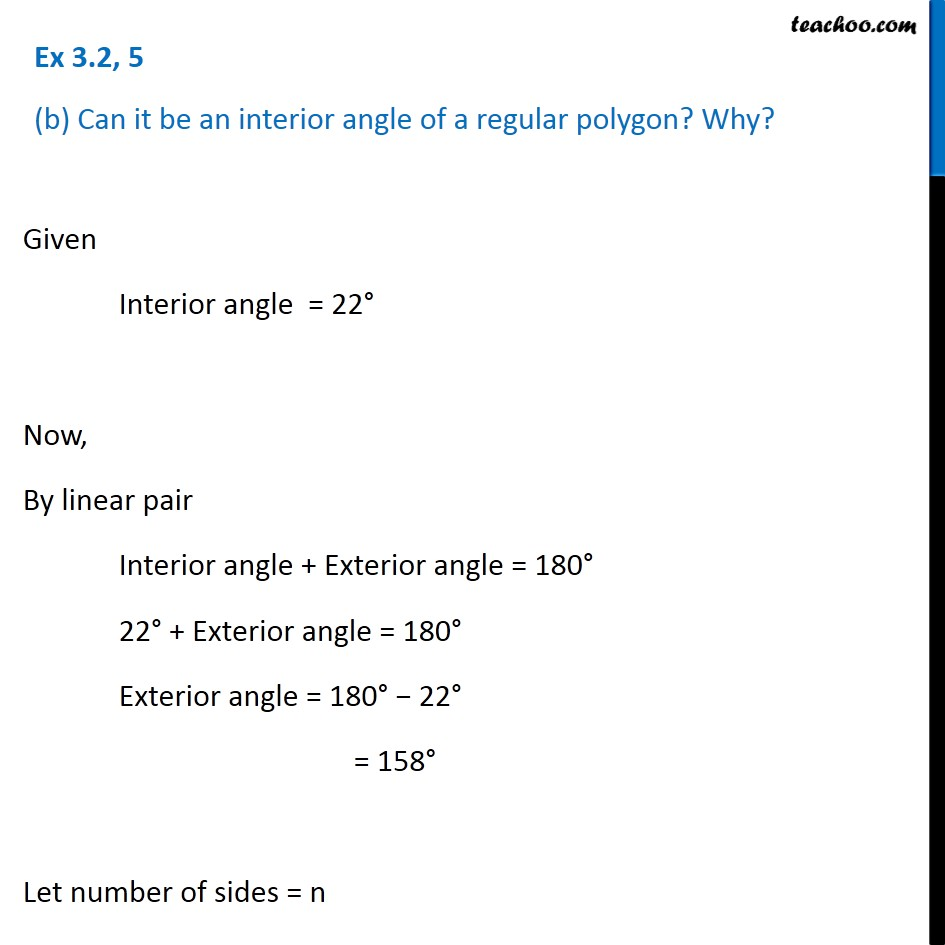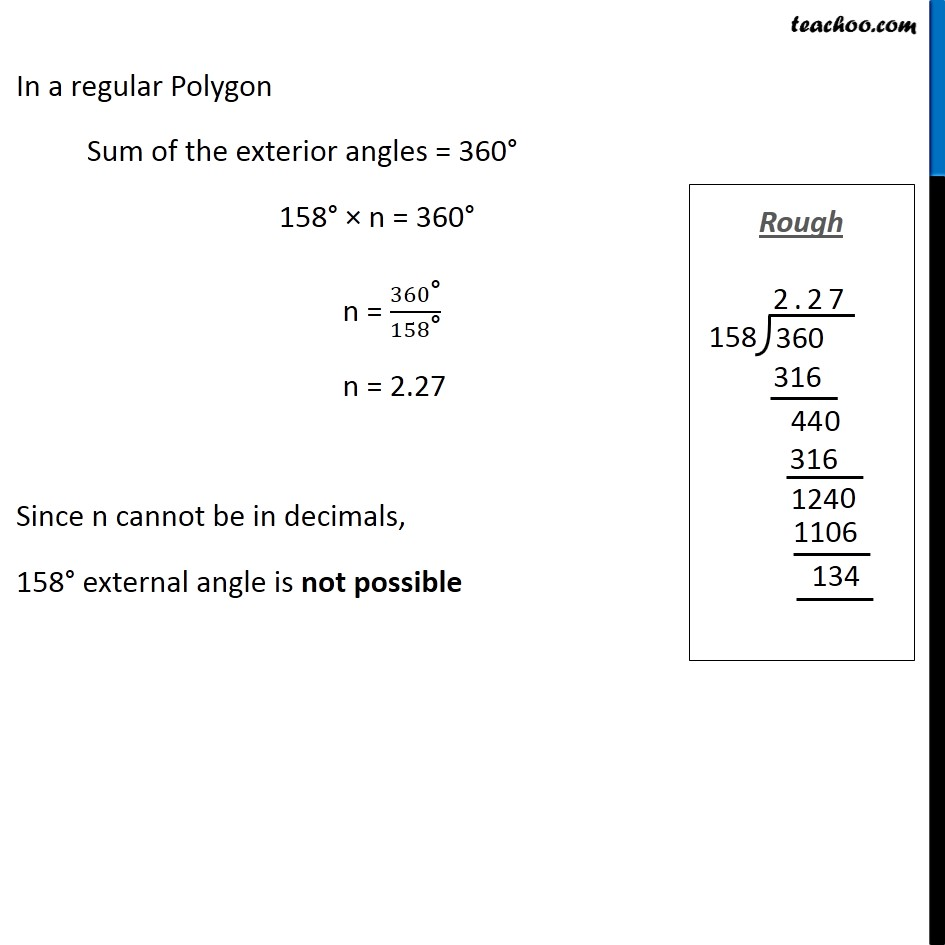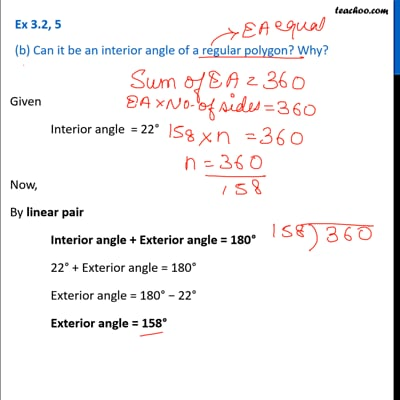Ex 3.2

Chapter 3 Class 8 Understanding Quadrilaterals
Serial order wiseThis video is only available for Teachoo black users

Learn in your speed, with individual attention - Teachoo Maths 1-on-1 Class

### Transcript

Ex 3.2, 5 (b) Can it be an interior angle of a regular polygon? Why? Given Interior angle = 22° Now, By linear pair Interior angle + Exterior angle = 180° 22° + Exterior angle = 180° Exterior angle = 180° − 22° = 158° Let number of sides = n In a regular Polygon Sum of the exterior angles = 360° 158° × n = 360° n = 360"°" /158"°" n = 2.27 Since n cannot be in decimals, 158° external angle is not possible